# Alpha vs Numeric: The Front Line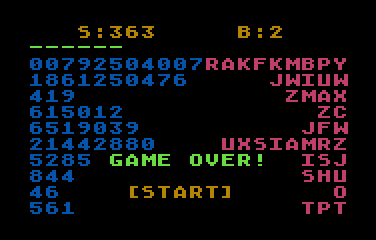This is a little game for the 2016 NOMAM's BASIC 10-liners Contest. This program fits in the PUR-120 category, and it was written using TurboBASIC XL 1.5 for the 8-bits ATARI XL/XE. Development started on 2016-02-12, and it took 4 days. The final version's date is 2016-02-21.

UPDATE: It obtained the 7th place of 22 entries in the category.

## Description

Alphabetics are in war against Numerics. Each army is sending their soldiers to the battles. As a mediator, you must call every soldier by their name and pick them out before the armies come in contact or one army come across the whole battlefield.

Each soldier you pick from the field gives you a point. You can only call soldiers that are in the front of each row. If there are many soldiers with the same name as the first in a line, you'll earn extra points, but if you call someone that is not in in the front line, extra soldiers would be send to the field.

You must pick 200 soldiers to declare a battle as draw, but new soldiers are sent more quickly as time runs, so you need to be fast enough. Every next battle will be faster and will begin with more soldiers in the field.

## Instructions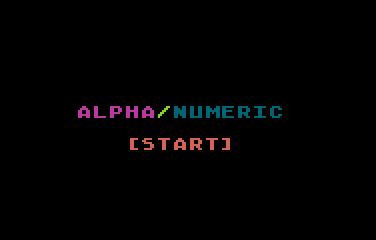Press START key to play.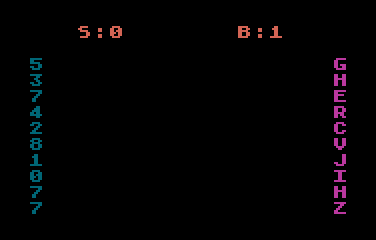The game starts with a group of letters and numbers. More letters and numbers will appear during the game, every time will be faster. Try to avoid both groups to meet in the middle of the screen.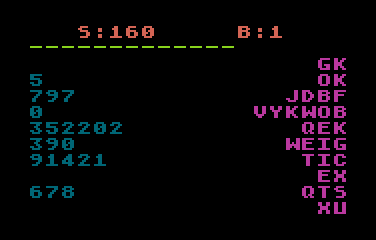Type the leftmost character from the alphabetic lines and the rightmost digit from the numbers lines to remove them. If you hit a wrong key, more chars will be added immediatelly. A progress bar will increase while characters are removed.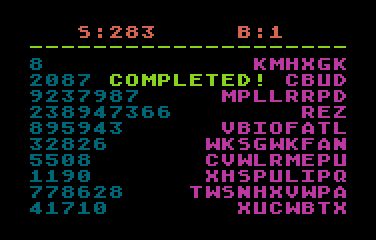When 200 characters are removed, the battle is over.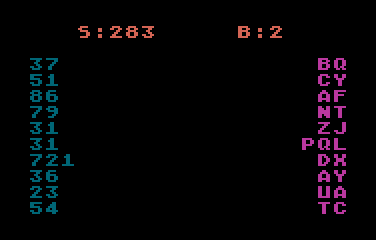Every new battle will start with more characters on both sides of the screen.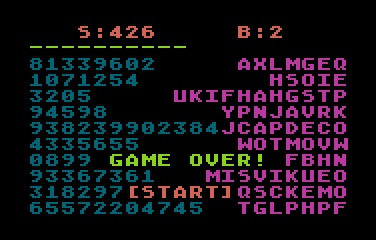When a number meets a letter, or one of them crosses the screen, tha game is over. Press START key to play again.

## Development of the game

After three 10-liners games written to be used with a joystick, I wanted to do something different... I thought about some kind of sliding items that must be captured or killed as fast as possible before they reach the other side of the screen, and a paddle was the chosen game controller... It should be like an horizontal Kaboom! style of game.

Before I could sit and write a prototype, I thought that if the sliding items are letters, they could be killed just by typing them. But it would be easy to tap over all the keyboard in a random way to kill if any of them matches, so a punishment should be applied.

After some other ideas, I decided that the playfield should have a row of letters and they would increase in length randomly, but only the first letter in the row should be killed, the other ones are only distractors. Pressing a letter that is not the first one on some line, the punish applies: extra letters immediately go into the playfield. More times you miss, an increased ammount of letters appear.

The last idea that it came into my mind was to include numbers, but appearing from the other side. More difficult, more challenge, more fun!

Then I sat down and started to program the proof of concept for that game. I started with one side only, and measure how many lines should be needed, because in the worst case, it should be doubled to include the numbers. Result: 4 lines and some bytes more. Of course, doubling the code wouldn't be practical and I needed to find some kind of formula to manage both sides at the same time with the same code. New count of BASIC lines: a working prototype of 5 lines and a half! That gave me more or less 3 lines to do some fine tunning of the speed up, and to add prizes, punishments, sounds and whistles.

I wrote this game in a free-form text editor in my PC, then parse it with a small tool (*) I wrote in perl language to generate a BASIC listing in ATASCII, with abbreviations and most of the unrequired spaces removed to save space and try to follow the 10-liners rules. For the test, I used Altirra, with an ATR with DOS and TurboBASIC XL in D1: and my development directory mounted as a virtual drive in D2:, so I only had to type E."D2:A.LST to load every new version.

(*) My little perl tool is very limited in functionalities, but I like it because it does what I need in the way I want. If you'd like to try one of these tools, I'd suggest DMSC's TurboBasic XL Parser Tool.

Get the ALPHANUM.ATR file and set it as drive 1 in a real Atari or emulator. Turn on the computer and the game should start after loading.

## The code

The abbreviated BASIC code in 10 lines with up to 120 chars each is the following: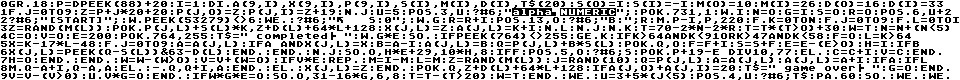The expanded BASIC listing is:

 ```GRAPHICS 18 P=DPEEK(88)+20 ``` Set up the screen as graphics mode 2 without the text window: 12 lines of 20 big chars, assigning 1 line for the score, 1 for the progress bar and 10 for the battlefield. P stores the starting memory address of the green progress bar. ```I=1 ``` Tokenized lines in TurboBASIC have a length restriction of 255 bytes. Each number requires 7 bytes in tokenized lines (1 byte for the token and 6 for BCD data), so a line with many numbers will reach the maximum internal length even if they are short in the listing. I decided to use 2 constants in variables: letter O for "zero" and I for "one". These use only 1 byte in the tokenized line. BTW, O doesn't need to be explicitely assigned, as TurboBASIC initializes every variable with zero at the start of the program. ```DIM A(9,I),X(9,I),P(9,I),S(I),M(I),D(I),T\$(20) ``` Reserves memory for the arrays for the game control. All of these are used to store the control variables for each of the armies. Unidimensional arrays has only 2 values, and bidimensional stores 2 values for each row of the battlefield. Note that the information about the soldiers that are in the field is not stored in arrays or other variables, with the exception of the leader of each row in X() and the number of soldiers in a row in A() just to speed-up the validation of a pressed key. ```S(O)=I S(I)=-I M(O)=10 M(I)=26 D(O)=16 D(I)=33 ``` S() is the direction in which each army walks.M() has the number of different soldiers of each army.D() is the "internal" name of the first of the soldier (as stored in screen memory). ```FOR J=0 TO 9 Z=P+J*20+20 P(J,O)=Z P(J,I)=Z+19 NEXT J ``` P() has the memory location of the starting point where ever row starts for each army. ```U=5 ``` U stores the number of the line in the screen where messages will be printed. Game name starts at 6th line (first line is numbered as 0). ```POSITION 3,U ? #6;"ALPHA/NUMERIC"; ``` Game title. This happens only once. ```DPOKE 731,1 ``` Disables the keyclick sound. ```WHILE I ``` Infinite loop. The following lines reinitializes variables for a new game. ``` N=O ``` Number of starting soldiers of each row for a battle. ``` G=I ``` Game is over when this changes to 0. ``` S=O ``` Initial score. ``` R=O ``` Number of the battle. ``` POSITION 6,U+2 ? #6;"[START]"; ``` Request for START key to continue. This appears 2 lines below the previous message. ``` WHILE PEEK(53279)<>6 WEND ``` Waits until START key is pressed. ``` ?#6;"} S:0"; ``` Clears the screen and prints the initial score. "}" is actually CHR\$(125), the Atari's clear screen symbol. ``` WHILE G ``` Main loop. Game is going on... First, initialize for a new battle: ``` R=R+I POSITION 13,O:?#6;"B:";R ``` Sets the battle number and print it on screen. ``` MOVE P-I,P,220 ``` Clears the progress line and the battlefield. This requires that the last byte of the score line been empty. ``` FOR K=0 TO N FOR J =0 TO 9 FOR L=0 TO I ``` Set up the initial screen for a battle: N+1 columns for every of the rows for both sides. ``` Z=RAND(M(L)) ``` Choses one possible soldier for a given army. ``` POKE P(J,L)+S(L)*K,Z+D(L)+64*L+128 ``` Finds the memory location for that soldier and places it there using the proper army colour. ``` X(J,L)=Z ``` X() has the first soldier in a row for a given army. ``` A(J,L)=K+I ``` A() has the length of the rows. ``` NEXT L NEXT J NEXT K ``` The first soldier in a row and the length of it is assigned many times, but the last ones remain for the battle. This is just to save code. ``` T=70-2*N-2*R T=T*(T>O)+30 W=T ``` Sets the initial value for the countdown before a new soldier is sent to the field. Higher the number of the battle, lower is the initial timer, but is has at least an initial value of 30. T will be decreased by 1 every time a new soldier is released, and W is the countdown to release a soldier, starting from current T value. ``` N=N+(N<5) ``` Increment the number of initial soldiers in a row at the beginning of a battle. This would never be more than 6 at each side, so the minimum clear battlefield width would be only 8 spaces! ``` C=O ``` This is the punishment counter. Every time you hit a wrong key, this increments by 1. It is reset to zero at the start of every battle. ``` V=O ``` Initializes the number of extra soldiers that will be sent to the battlefield at the same time as a punishment or because the counterclock ends. ``` E=200 ``` This is the initial number of required hits to finish a battle. ``` POKE 764,255 ``` Need to clear the register for a possible pressed key before the start of the battle. ``` T\$=" COMPLETED! " ``` Default message to be displayed when a battle ends. ``` WHILE G * E ``` This is the battle loop. A battle has finished after the required hits is completed or when the game is over because the armies got in touch. ``` SOUND ``` Turn off the sounds that started on the previous loop. ``` IF PEEK(764)<>255 ``` Check if a key was pressed. ``` GET K IF K>64 AND K<91 OR K>47 AND K<58 ``` If so, get the ASCII value of it, and check if it was a letter or a number. Other values are being discarded. ``` F=O ``` Initializes the counter of matches for that key. ``` L=K>64 ``` Check if it was a letter or a number, and set L as the army index. ``` X=K-17*L-48 ``` Calculates the value of the soldier based on it's number, not the ATASCII value on the screen. ``` FOR J=0 TO 9 ``` Search for that number in the list of first soldier at the current army. ``` A=A(J,L) IF A AND X(J,L)=X ``` If this row has soldiers and the first one is the required one... ``` B=A-I A(J,L)=B ``` decrement the number of soldiers in that row, ``` Q=P(J,L)+B*S(L) POKE Q,O ``` calculate the screen position of that soldier, and remove it, ``` F=F+I ``` increment the hits counter, ``` S=S+F ``` increase the score based on the number of hits (with bous if it appears many times), ``` E=E-(E>O) ``` decreases the pending number of required hits in, ``` H=I ``` and set the "found flag" for the pitch and distortion of the sound for the keypress. ``` IF B X(J,L)=PEEK(Q-S(L))&63-D(L) ENDIF ``` If the line stil have soldiers, saves the number of the new one in front of it. ``` ENDIF NEXT J SOUND O,H*E+29,10*H,8 ``` Start a sound based on the result of the search. The pitch is based on the number of remaining hits: a higher pitch, a lower number of pending hits. But a miss turn the sound in a scratch! ``` IF F POSITION 5,O ? #6;S POKE P+19 - E DIV 10,77 ``` If found, print the new score and increment the progress bar: one step represents 10 hits. ``` ELSE C=C+I V=C ENDIF ``` If not found increment the punisment counter in C and set with that value the number of extra soldiers to include immediately in the field. ``` H=O ENDIF ENDIF ``` Reset the "found flag" for the next iteration. ``` W=W-(W>O) ``` Decrement the countdown if it is a positive value. ``` V=V+(W=O) ``` Sets the total number of soldiers to add: the punishment amount plus one if the countdown reached zero. ``` IF V * E ``` If there are pending hits and soldiers are required, do the routine to add them. ``` REPEAT M=I-M L=M ``` Add soldiers to the battlefield, selecting the army from other side according to the previous addition. ``` Z=RAND(M(L)) ``` Randomly select a soldier for the given army. ``` J=RAND(10) ``` Select a row where it will be added, also randomly. ``` Q=P(J,L) ``` Find the memory location of the screen position where a row starts growing. ``` A=A(J,L) A(J,L)=A+I ``` Get the number of soldiers (the length) of that row and increase it by one. ``` IF A IF L MOVE Q-A+I,Q-A,A ELSE -MOVE Q,Q+I,A ENDIF ``` If there was at leas one soldier in the row, the row must advance one step to make a place for the new soldier. Based on the side of the army, a MOVE or -MOVE (reverse MOVE) instruction must be performed. ``` ELSE X(J,L)=Z ENDIF ``` If the row was empty, no move is required, but the new soldier has to be assigned as the new leader in front of the line. ``` POKE Q,Z+D(L)+64*L+128 ``` Place the new soldier in the field, with its required shape and colour. ``` IF A(J,O)+A(J,I)=20 T\$=" GAME OVER! " G=O ENDIF ``` Check if the enlarged line meets the line of the other army, or if it reached the other side of the screen. If it does, the game is over. Clears the G game flag and changes the message for the end of the battle. ``` V=V-(V>0) ``` Decrease the number of required soldiers at this time. ``` UNTIL V * G = O ENDIF ``` Loop again this routine until no more soldiers are required or the game is over. ``` IF W * G * E = O SOUND O,31-16*G,6,8 ``` Play a sound when the countdown finishes, the game is over or the battle is over. This sound is turned off immediately at the begining of the loop if neither the round or the game is over, giving a simple "tick" sound, otherwise, the sound will be a sort of notification: there is a low pitch is lower when the game is over and a high pitch when the battle ends. ``` T=T-(T>20) W=T ENDIF ``` Restart the countdown counter, but the maximum value is one less than the previous. This way, things happen more frequently as time goes... ``` WEND ``` End of the battle loop. ``` U=3+5*(J<5) POSITION 4,U ?#6;T\$ ``` Print the message. The position of the message depends on the las line where was added a soldier. This is important, because if the armies met in the bottom half of the screen, the end of game message appears in the upper half, and viceversa. ``` PAUSE 60 SOUND ``` Waits for a moment after the battle ends. During this time, the "tick" sound becames a notification bell. ``` WEND ``` End of the current game loop. ```WEND ``` Restart...